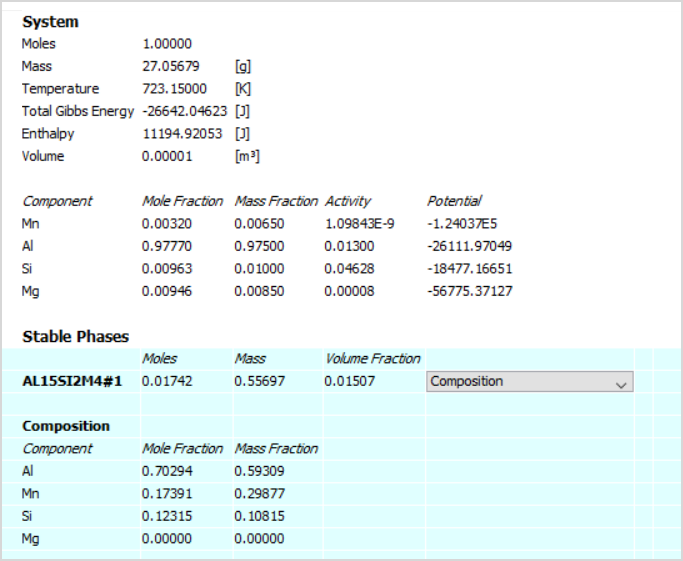# Equilibrium and Non-equilibrium Calculations

## Equilibrium Calculator

Calculate phase diagrams, stable phases, and more using this fundamental calculator included in all Thermo-Calc installations.

The Equilibrium Calculator is a fundamental calculator within Thermo-Calc that allows users to easily set up and perform equilibrium and non-equilibrium calculations under a range of different conditions. The calculator is used in a range of different situations, including when calculating phase diagrams.  It is included in all Thermo-Calc installations.

## Flexible Conditions

The Equilibrium Calculator in Thermo-Calc offers user’s large flexibility in terms of the different conditions that can be specified for the calculation. Examples of conditions (state variables) that can be set include: temperature, pressure, amount of component (for example mass, mass-percent, moles, mole-percent), amount of phase (mass, moles, volume), amount of component in a phase, chemical potential of component, activity of component, enthalpy, entropy, and more.

## Four Calculation Types

The Equilibrium Calculator includes four calculation types that allow users to make a range of calculations: single equilibrium, one axis, grid, and phase diagram, as explained below.

## Single Equilibrium

This calculation type allows you to calculate the equilibrium for a specific set of conditions. The results of the calculation are provided in the form of a table, which shows the stable phases that are predicted to form under those conditions and the composition of those phases.

The table shows partial results of an equilibrium calculated at 450°C for Al-1Si-0.85Mg-0.65Mn (AA6082). Stable phases not shown in the image are FCC_A1 and MG2SI_C1.## One Axis

This calculation type allows users to vary (or step in) one of the conditions specifying equilibrium. In this way, a series of equilibrium calculations is made where one of the conditions is changing, in steps, from one value to another. The results can be provided in a table or as a plot, as is the case for the example, which shows the volume-fraction of all stable phases present in a commercial Al-alloy, as a function of temperature.

A range of other properties can be plotted in a similar way, for instance composition of phases, heat capacity, density, and many more.

The plot shows a one-axis calculation of volume-fraction of stable phases as a function of temperature for Al-5.6Zn-2.5Mg-1.6Cu-0.2Si-0.15Mn (AA7075).## Grid

When selecting this calculation type, two conditions specifying the equilibrium are varied with equidistant steps. The result is provided as a plot, such as in the example here showing the viscosity in an Al-SI-Mg-Mn melt, just above the liquidus temperature.

The plot shows a grid calculation of dynamic viscosity calculated at 680°C for Al-1Si-yMg-xMn alloys.## Phase Diagram

In this calculation type, two conditions are specified and varied, just as with a grid calculation, but in this case phase boundaries (or zero phase fraction lines) are tracked and followed to generate a phase diagram. The result is provided as a plot, as shown in the example, where the Si content is varied on the x-axis and temperature on the y-axis.

The plot shows a phase diagram, also known as an isoplethal section, for Al-xSi-0.85Mg-0.65Mn.## Phase Diagram in Isothermal Conditions

Users can generate another type of phase diagram by varying the amounts of two elements at a constant temperature (isothermal conditions). Such an example is shown here, which is a phase diagram calculated at 1100°C for a ternary Fe-Cr-Mo system.

The plot shows a ternary phase diagram, also known as an isothermal section, for Fe-Cr-Mo at 1100°C.## Databases

The Equilibrium Calculator uses the same thermodynamic and properties databases used for all Thermo-Calc calculations. However, if you want to calculate diffusion coefficients, a mobility database is also required. The mobility database contains information about the atomic mobility of individual components in relevant phases, and each mobility database corresponds to a specific thermodynamic and properties database. Information about our broad range of databases can be found in the database section.

## Availability

Calculating a Single-point Equilibrium

Stepping in Temperature in the Fe-C System

Carbide Driving Force Heat Map

Fe-C Phase Diagrams